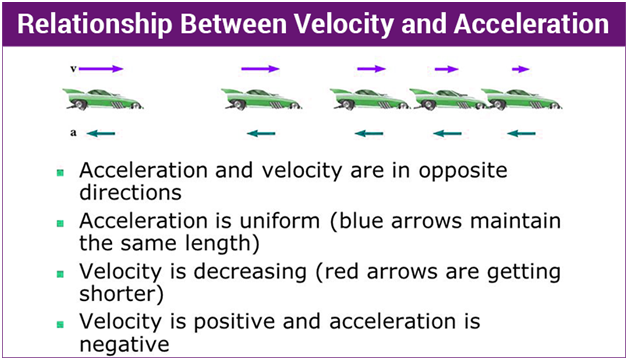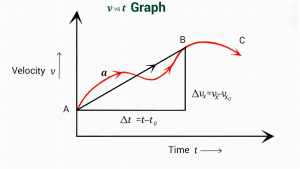# Difference Between Velocity and Acceleration

0
369Velocity and acceleration seem similar, but they are quite different from each other. You must understand both the terms if you want to learn the difference between them. In this post, we will uncover the major differences between velocity and acceleration by explaining both terms comprehensively. So, stick to the topic till the end to get familiar with the dissimilarities between velocity and acceleration effectively.

Both velocity and acceleration are the two major terminologies of physics and are associated with the motion of an object. Suppose an object moves from its fixed position concerning external force, then the object is considered to have undergone motion like the earth is in motion, etc.

There are four main types of motion such as rotary, oscillatory, reciprocate, and linear. In all these types of motion, an object changes its position over time.## Speed

Speed is a measurement of distance travel over time. The Speed is a scalar quantity.

### Scalar quantity

A scalar quantity is the measure of a dimensional quantity, such as only its magnitude, for example, temperature and mass.

### Vector quantity

Vector quantity is the measure of a two-dimensional quantity, such as its magnitude and direction, such as velocity and acceleration.

### Velocity difference between velocity and acceleration

Velocity is the rate of change of position of an object over time; for example, a car moves at a speed of 74 miles per hour. Velocity is a vector quantity. We all know that a change in position with direction is papular as displacement. So, the equation of velocity is v=change in position /change in time. The standard international (S.I) unit of velocity is meter per second m/s.

Commonly we know velocity as speed, but in physics, speed and velocity are two different terminologies because speed is a scalar quantity and velocity is a vector quantity.

Different types of velocity are uniform velocity, variable velocity or non-uniform velocity, average velocity, and instantaneous velocity. Velocity position value can be positive, negative, or sometimes zero.

## Acceleration difference between velocity and acceleration

Now, you might have understood the term velocity; let’s move towards acceleration. In everyday conversation, we used the word acceleration in terms of speed up. For example, accelerating the car means speeding up the car.

But in physics, acceleration means a change in speed and direction.

So the definition of acceleration is the rate at which the velocity of an object changes in terms of speed and direction. For example, if the object is moving in a straight line, it accelerate if its speed changes either increase or decrease. And suppose an object is moving in a circular motion. In that case, it say to be accelerated even if its speed is constant, neither increasing nor decreasing, because the direction of an object is continually changing.

Any change in the velocity of an object will result in acceleration. But people think that the only increase in speed is acceleration which is not true. Acceleration is also a vector quantity.

Its S.I unit is a meter per second square m/s2. Equation of acceleration is

a= change in velocity/change in time.

There are two main types of acceleration: average acceleration determine over a long interval of time, and instantaneous acceleration is determined over a short interval of time.